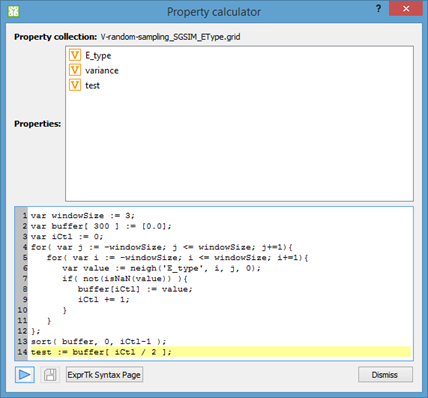# vtkImageMathematics for Image with Complex Data

Hello,

I am writing code in C++ to do some mathematical operations on matrices that contain complex numbers. I am using vtk to accomplish this, primarily because the data I am working with needs to be resliced, resized, transformed with FFT, etc. The vtkImageReslice and other image-related classes work nicely for this.

One operation I am trying to perform on a vtkImageData object is exponentiation. In other words, I want to do exp(X) where X is a vtkImageData object. The image data consists of complex numbers which I have stored in two components in the vtkImageData object. vtkImageMathematics class has an exp() operation, but I don’t think it was designed to work with complex numbers. Is this correct? For complex numbers in the form z = x + iy, the complex exponential is defined as: e^z = e^x(cosy + isiny).

To accomplish this with vtkImageMathematics and other classes, the code looks kind of ugly. I must be doing something wrong. Is there a better way? Is vtk even the right tool to be using? This must be accomplished in C++, not Python. So numpy is not an option.

Michelle

``````// filters for extracting real and imaginary components of complex image
vtkSmartPointer<vtkImageExtractComponents> extractRealFilter = vtkSmartPointer<vtkImageExtractComponents>::New();
extractRealFilter->SetComponents(0);
vtkSmartPointer<vtkImageExtractComponents> extractImaginaryFilter = vtkSmartPointer<vtkImageExtractComponents>::New();
extractImaginaryFilter->SetComponents(1);

// extract the real component from my complex numbers image
extractRealFilter->SetInputData(complexImage);
extractRealFilter->Update();

// e^x
vtkSmartPointer<vtkImageMathematics> exponentFilter = vtkSmartPointer<vtkImageMathematics>::New();
exponentFilter->SetOperationToExp();
exponentFilter->SetInput1Data(extractRealFilter->GetOutput());
exponentFilter->Update();

// extract the imaginary component from my complex number image
extractImaginaryFilter->SetInputData(complexImage);
extractImaginaryFilter->Update();

// cosy
vtkSmartPointer<vtkImageMathematics> cosFilter = vtkSmartPointer<vtkImageMathematics>::New();
cosFilter->SetOperationToCos();
cosFilter->SetInput1Data(extractImaginaryFilter->GetOutput());
cosFilter->Update();

// siny
vtkSmartPointer<vtkImageMathematics> sinFilter = vtkSmartPointer<vtkImageMathematics>::New();
sinFilter->SetOperationToSin();
sinFilter->SetInput1Data(extractImaginaryFilter->GetOutput());
sinFilter->Update();

// e^x * cosy
vtkSmartPointer<vtkImageMathematics> cosMultFilter = vtkSmartPointer<vtkImageMathematics>::New();
cosMultFilter->SetOperationToMultiply();
cosMultFilter->SetInput1Data(exponentFilter->GetOutput());
cosMultFilter->SetInput2Data(cosFilter->GetOutput());
cosMultFilter->Update();

// e^x * siny
vtkSmartPointer<vtkImageMathematics> sinMultFilter = vtkSmartPointer<vtkImageMathematics>::New();
sinMultFilter->SetOperationToMultiply();
sinMultFilter->SetInput1Data(exponentFilter->GetOutput());
sinMultFilter->SetInput2Data(sinFilter->GetOutput());
sinMultFilter->Update();

// fill a new vtkImageData object with the resulting real and imaginary components
vtkSmartPointer<vtkImageData> expImage = vtkSmartPointer<vtkImageData>::New();
expImage->SetSpacing(1, 1, 1);
expImage->SetExtent(0, complexImage->GetDimensions() - 1, 0, complexImage->GetDimensions() - 1, 0, 0);
expImage->AllocateScalars(VTK_DOUBLE, 2);  // complex numbers, two components
for (int row = 0; row < complexImage->GetDimensions(); row++)
{
for (int col = 0; col < complexImage->GetDimensions(); col++)
{
double* realPix = static_cast<double*> (cosMultFilter->GetOutput()->GetScalarPointer(col, row, 0));
double* imaginaryPix = static_cast<double*> (sinMultFilter->GetOutput()->GetScalarPointer(col, row, 0));
double* complexExpPix = static_cast<double*> (expImage->GetScalarPointer(col, row, 0));
complexExpPix = realPix;
complexExpPix = imaginaryPix;
}

}``````

Hi, Michelle,

The more complex the operation, the more complex the code. That is the way `vtkImageMathematics` seems to work according to its documentation: do one operation per pass.

In my project, I used ExprTk (.:: C++ Mathematical Expression Library (ExprTk) - By Arash Partow ::.) which is a header library (easy to add to your project). ExprTk is an expression parser that allows users to enter the mathematical expressions they want, regardless of complexity and your code doesn’t become ugly or difficult to maintain. I’ve been using this for quite a while and I found it’s performance quite good for a script engine, which is about half as fast as native code running in a single thread. IMO, it’s a fair balance between performance and flexibility. It used for geologic modeling, doing calculations for tens of millions of cells in less than a second.

If you’re interested in adding a calculator engine to your project, you can take a look at the classes here: gammaray/calculator at master · PauloCarvalhoRJ/gammaray · GitHub (they are easily transplantable to another project, provided you implement an interface). The class that does the parsing and calculation is gammaray/calcscripting.cpp at master · PauloCarvalhoRJ/gammaray · GitHub .

regards,

Paulo

Hi Paulo,
Thank you very much for your reply. And thanks for introducing me to ExprTk and your calculator engine.
Best wishes,
Michelle

Hi, Michelle,

This is a screen shot of the dialog:The entered script (a median filter) is executed for each grid cell. You can provide users with practically unlimited calculation possibilities without resorting to binding to a complete scripting environment (Python, Julia, R, etc.) which is overkill for such task and more technically difficult to do.

regards,

Paulo